Failure Time Distribution

Let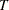be a nonnegative random variable that represents the failure time of an individual from a homogeneous superpopulation. The survival distribution function (also known as the survivor function) ofis written as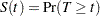A mathematically equivalent way of specifying the distribution ofis through its hazard function. The hazard function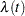specifies the instantaneous failure rate at. Ifis a continuous random variable,is expressed as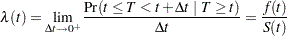where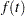is the probability density function of.